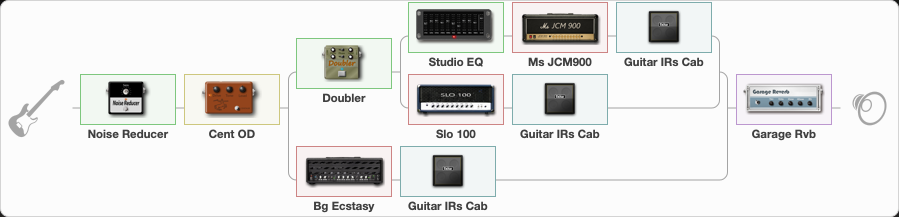# Megadeth - Rust in peace

Discussion in 'ToneLib-GFX presets' started by grigoriy0192873, Jan 19, 2023.

1. ### grigoriy0192873Active Member

Preset name: Rust in peace

Effects chain:Effect: "Noise Reducer" (Dynamics / Filter), active - "yes"
{
"Sens" = 80
"Mode" = Soft
}

Effect: "Cent OD" (Overdrive / Distortion), active - "yes"
{
"Drive" = 50
"Tone" = 70
"Level" = 70
}

Effect: "Splitter" (Dynamics / Filter)
{
"A-Bypass" = Off
"A-Pan" = -25
"A-Level" = 55
"B-Bypass" = Off
"B-Pan" = 25
"B-Level" = 55
"Width" = 0

'A' branch:
{

Effect: "Doubler" (Dynamics / Filter), active - "yes"
{
"A-Dly" = 75
"A-Inv" = Off
"A-Level" = 75
"B-Dly" = 50
"B-Inv" = Off
"B-Level" = 50
}

Effect: "Splitter" (Dynamics / Filter)
{
"A-Bypass" = Off
"A-Pan" = -25
"A-Level" = 55
"B-Bypass" = Off
"B-Pan" = 25
"B-Level" = 55
"Width" = 0

'A' branch:
{

Effect: "Studio EQ" (Dynamics / Filter), active - "yes"
{
"31 Hz" = 0
"62 Hz" = 0
"125 Hz" = 0
"250 Hz" = 0
"500 Hz" = 0
"1 kHz" = 0
"2 kHz" = 0
"4 kHz" = 0
"8 kHz" = 0
"16 kHz" = 0
"Level (dB)" = 0
}

Effect: "Ms JCM900" (Amp simulators), active - "yes"
{
"Gain" = 60
"Bass" = 40
"Middle" = 60
"Treble" = 60
"Presence" = 60
"Master" = 50
"Level (dB)" = 0
}

Effect: "Guitar IRs Cab" (Cabinets), active - "yes"
{
"Model" = Marshall 1960A (4x12")
"Mic Position" = Middle
"Mic Distance" = Near
"Low Cut (Hz)" = 60
"Hi Cut (kHz)" = 20.0
"Mix" = 100
"Level (dB)" = 0
}
}
'B' branch:
{

Effect: "Slo 100" (Amp simulators), active - "yes"
{
"Gain" = 51
"Bass" = 100
"Middle" = 40
"Treble" = 60
"Presence" = 81
"Master" = 50
"Level (dB)" = 0
}

Effect: "Guitar IRs Cab" (Cabinets), active - "yes"
{
"Model" = Marshall 1960b (4x12")
"Mic Position" = Middle
"Mic Distance" = Near
"Low Cut (Hz)" = 60
"Hi Cut (kHz)" = 20.0
"Mix" = 100
"Level (dB)" = 0
}
}
}
}
'B' branch:
{

Effect: "Bg Ecstasy" (Amp simulators), active - "yes"
{
"Gain" = 80
"Bass" = 30
"Middle" = 50
"Treble" = 80
"Presence" = 80
"Master" = 50
"Level (dB)" = 0
}

Effect: "Guitar IRs Cab" (Cabinets), active - "yes"
{
"Model" = Marshall JCM2000 (4x12")
"Mic Position" = Middle
"Mic Distance" = Near
"Low Cut (Hz)" = 60
"Hi Cut (kHz)" = 20.0
"Mix" = 100
"Level (dB)" = 0
}
}
}

Effect: "Garage Rvb" (Reverberation), active - "yes"
{
"Time" = 5.2
"PreDelay" = 46
"LoDamp" = 0
"HiDamp" = 30
"Mix" = 10
}

Note: You will need to download and install the ToneLib-GFX software to use the preset.